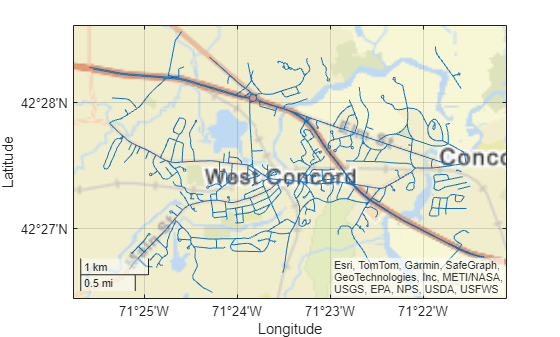maplineshape

Line shape in planar coordinates

Description

A maplineshape object represents a line or multiline in planar coordinates. A multiline is an individual line shape that contains a set of separate lines.

To represent a line or multiline in geographic coordinates, use a geolineshape object instead.

Creation

To create maplineshape objects, either:

• Import line data in planar coordinates as a geospatial table using the readgeotable function, and then query the Shape variable of the table.

• Use the maplineshape function (described here).

Description

example

shape = maplineshape(x,y) creates a maplineshape object or array of maplineshape objects with vertices at the specified x- and y-coordinates. The sizes of x, y, and the maplineshape object array shape match.

Input Arguments

expand all

x-coordinates, specified as a numeric vector or a cell array of numeric vectors.

• Create a line by specifying a vector, such as [65 62 53 66].

• Create a multiline by specifying a vector and including line breaks as NaN values, such as [55 34 18 NaN 14 19 42 26].

• Create an array of lines and multilines by specifying a cell array of vectors, such as {[55 34 18],[14 19 NaN 42 26 31]}.

The NaN values in x must correspond to the NaN values in y.

The size of x must match the size of y. For cell arrays, the size of the vector in each cell of x must match the size of the vector in the corresponding cell of y.

Data Types: double | cell

y-coordinates, specified as a numeric vector or a cell array of numeric vectors.

• Create a line by specifying a vector, such as [4 59 121 98].

• Create a multiline by specifying a vector and including line breaks as NaN values, such as [78 56 63 NaN 83 106 104 126].

• Create an array of lines and multilines by specifying a cell array of vectors, such as {[78 56 63],[83 106 NaN 104 126 131]}.

The NaN values in x must correspond to the NaN values in y.

The size of x must match the size of y. For cell arrays, the size of the vector in each cell of x must match the size of the vector in the corresponding cell of y.

Properties

expand all

Number of line parts, returned as an array of nonnegative integers.

For a maplineshape scalar, the value of NumParts is 1 when the maplineshape object represents a single line and more than 1 when the object represents a multiline.

For a maplineshape array, the size of NumParts matches the size of the array.

Data Types: double

Geometric type, returned as "line".

Data Types: string

Coordinate system type, returned as "planar".

Data Types: string

Projected coordinate reference system (CRS), specified as a projcrs object. A projected CRS consists of a geographic CRS and several parameters that are used to transform coordinates to and from the geographic CRS.

Examples

collapse all

Import a shapefile containing a network of road segments in Concord, MA as a geospatial table. The shapefile represents the road segments using lines. Get information about the fourth line by querying the Shape variable of the table.

GT.Shape(4)
ans =
maplineshape with properties:

NumParts: 1
Geometry: "line"
CoordinateSystemType: "planar"
ProjectedCRS: [1x1 projcrs]

mapshow(GT)Create an individual line as a maplineshape scalar. Specify the projected CRS as World Equidistant Cylindrical, which has the EPSG authority code 4087.

x = [4 59 121 98];
y = [65 62 53 66];
lineshp = maplineshape(x,y);

p = projcrs(4087);
lineshp.ProjectedCRS = p
lineshp =
maplineshape with properties:

NumParts: 1
Geometry: "line"
CoordinateSystemType: "planar"
ProjectedCRS: [1x1 projcrs]

Create a multiline as a maplineshape scalar.

x = [78 56 63 NaN 83 106 104 126];
y = [55 34 18 NaN 14 19 42 26];
multiline = maplineshape(x,y);
multiline.ProjectedCRS = p
multiline =
maplineshape with properties:

NumParts: 2
Geometry: "line"
CoordinateSystemType: "planar"
ProjectedCRS: [1x1 projcrs]

Create one individual line and one multiline as a 1-by-2 maplineshape array.

x = {[78 56 63],[83 106 NaN 104 126 113]};
y = {[55 34 18],[14 19 NaN 42 26 37]};
lineMultiline = maplineshape(x,y);
lineMultiline.ProjectedCRS = p
lineMultiline=1×2 object
1x2 maplineshape array with properties:

NumParts: [1 2]
Geometry: "line"
CoordinateSystemType: "planar"
ProjectedCRS: [1x1 projcrs]

Topics

Introduced in R2021b

Mapping Toolbox Documentation